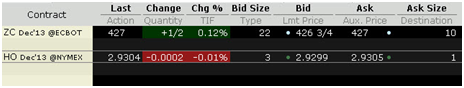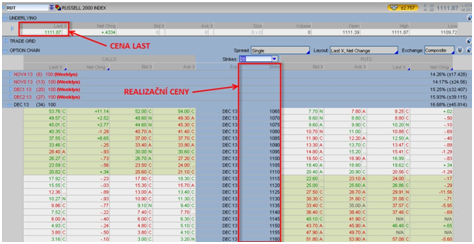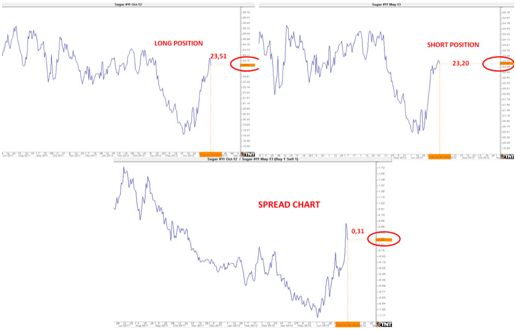If you are at least a little familiar with exchange trading, you've surely already come across the term spread. It is a frequently used term which, however, occurs in various contexts and therefore represents several different phenomena. As a result, there is often confusion regarding the spread interpretation and it is also often used in wrong contexts. Therefore, let's have a look at this term a little closer.

The essence of the term spread is the same in all trading contexts - it always represents a price range. In other words, it is a difference between two price values.

#### Price Range between BID and ASK

The most common use of spread in trading relates to the difference between the bid and ask prices.Let's demonstrate this on two examples. In the picture you can see a screenshot from the Interactive Brokers trading platform. On the first row there is a contract of corn with expiration in December 2013. As you can see, the current BID price is \$426.75 and the current ASK price is \$427. The spread between the two values ​​is thus \$427 - \$426.75 = \$0.25.

As a second example there is a contract of heating oil. In this case, the BID price is \$2.9299 and the ASK price is \$2.9305. The price range, i.e. spread, is therefore \$0.0006.

These were the two examples of the most common use of the term spread. However, this term can also be used in many other contexts.

Another most frequent use of the term spread relates to option trading. The most basic meaning of the spread in options trading is the difference, i.e. the price range, between the spot price (market price, i.e. the price for which the underlying asset can be instantly bought or sold) and the strike price of the underlying asset.In the picture you can see a screenshot from the ThinkorSwim (TOS) trading platform. The LAST price of the Russell 2000 index was \$1,111.87 and the displayed strike prices range between \$1,065 and \$1,160.  In this case, the spread would equal to the difference between the spot price of the index (i.e. market price at which it is possible to immediately open the trading position) and the selected strike price. Let’s assume that the spot price is \$1,111.87 and the strike price is \$1,130. The spread value will therefore equal to \$18.13. However, this is not a typical use of the term spread in option terminology.

The most often use of the spread term in option trading relates to combination of long and short positions of options of the same type (i.e. put or call) on the same underlying asset, but with different strike prices and different expirations. The spread in this case represents the price range between the two strike prices. There are three types of these spreads in option trading:

• Vertical spread (price spread, money spread) is a combination of long and short positions of the same type of options (call or put) with different strike prices. The current spot price of the underlying asset lies between the two strike prices.
• Horizontal spread (time spread, calendar spread) is a combination of long and short positions of the same type of options (call or put) with the same strike prices, but with different expirations.
• Diagonal spread is a combination of both of the above spreads. It means that in this case the options differ in strike prices as well as in expiration dates.

Another area in which we can work with spreads is a futures contracts trading. In this context we can often come across the term commodity spread, although this term is somewhat misleading. It is because it does not only relate to trading commodities, but to almost all types of underlying assets traded as futures. The proper term should therefore be futures spreads.

Also in case of commodity spreads this term represents a price range. Futures spreads are, like in option trading, based on the principle of a combination of long and short positions. In this case, we distinguish the following 4 types of spreads:

• Interdelivery (intramarket, calendar) spread is a combination of long and short positions (i.e. a simultaneous opening of long and short positions) in the same market, but in different expiration months. As an example we can mention opening of a long position in sugar with expiration in October 2012 at the price of \$23.51 and a simultaneous opening of a short position in sugar with expiration in May 2013 at the price of \$23.20.The charts above display the chart of sugar with expiration in October 2012 and next to it the chart of sugar with expiration in May 2013. Below the two charts there is so called spread chart which shows increase/decrease of the price range between long and short positions in sugar futures contracts with expirations in October 2012 and May 2013.

The spread in this case is \$23.51 – \$23.20 = \$0.31.

• Intermarket spread is a combination of long and short positions of the same commodity traded at two different exchanges. For example purchase of a December corn on the Chicago Board of Trade (CBOT) and a simultaneous sale of a December corn on the Kansas City Board of Trade (KCBT). It is of course also possible to open positions with different expiration months.
• Intercommodity spread is a combination of long and short positions in different commodity markets. For example, purchase of a corn contract with expiration in December 2013 and a simultaneous sale of wheat with the same expiration month. Also here it is possible to combine different expiration months.
• Intracrop spread relates only to grains. It is a combination of long and short positions within the same harvest period.

### You might also be interested in